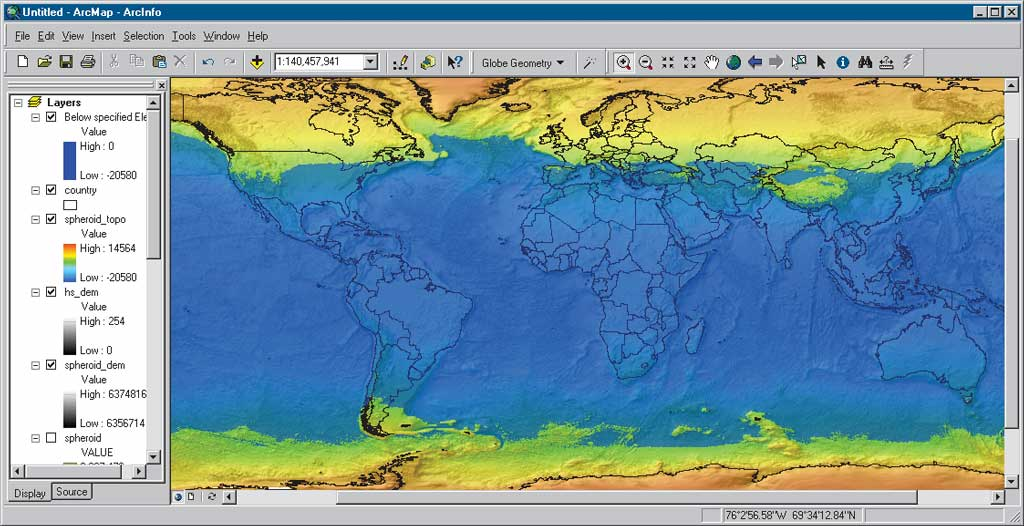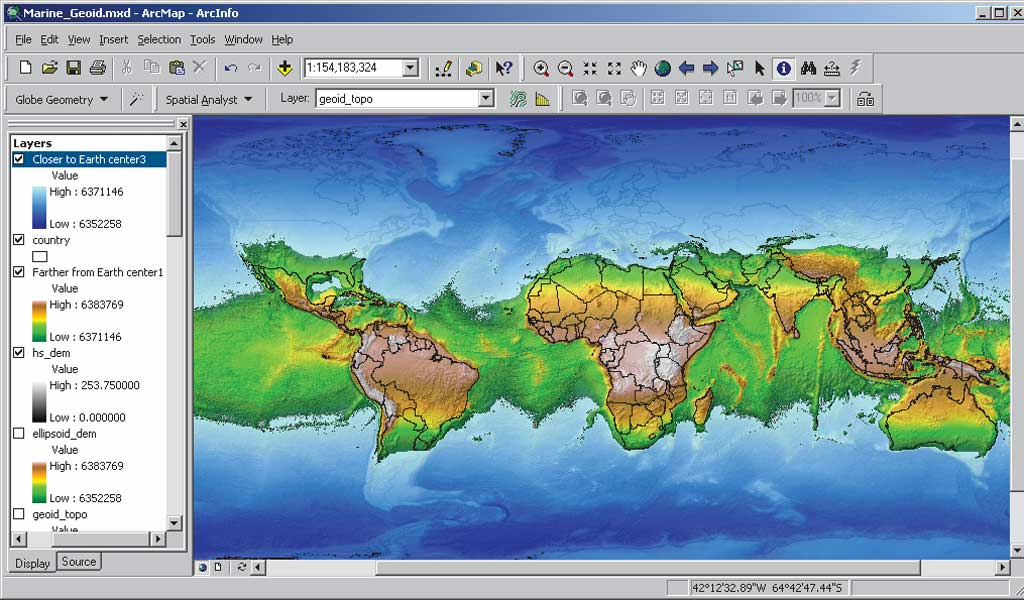# Gravitation field of the earth

## Recommended Posts

Why is the gravitational field of the earth is larger when one is located at the equator than located at the poles?

I know that rotation accounts for it, but why does rotation affect the earth's field strength? It doesn't make sense to me when we put a stationary object just above the earth relative to the sun, the gravitational field of the object changes a large amount when compared to the situation that it is in contact, rotating on the earth.

##### Share on other sites

Why is the gravitational field of the earth is larger when one is located at the equator than located at the poles?

I know that rotation accounts for it, but why does rotation affect the earth's field strength? It doesn't make sense to me when we put a stationary object just above the earth relative to the sun, the gravitational field of the object changes a large amount when compared to the situation that it is in contact, rotating on the earth.

Rotation doesn't affect gravity (assuming we're not getting into heavy-duty general relativity here), though it will affect your perception of gravity, since we tend to equate the normal force exerted on us with weight — this is what a bathroom scale actually measures.

Two things can come into play, depending on what you're looking at:

Because of the rotation, there is an equatorial bulge, meaning that you are further from the equator. There's a little more mass underneath you, but the net effect is that gravity is actually less at the equator than at the pole.

Objects in orbit are said to be weightless, but in fact do feel a gravitational force. The confusion is that an object moving in a circular orbit need to be accelerated at v^2/r (the centripetal acceleration), and if this is accounted for by gravity, then there is no other force on the object to be felt and perceived as gravity — it is in freefall. On the surface of the earth, we are not in orbit, but there is rotation, meaning that we need a smaller normal force to give us the value of centripetal force necessary to keep us moving in a circle about the earth's axis. Smaller normal force means the perceived gravity is less.

An object above the equator, not rotating with the earth, would feel heavier, but it's not because the gravity has changed. An object on a nonrotating planet would be heavier, because of the absence of the deformation.

##### Share on other sites

My book said that the gravitational field strength for an object at the equator is smaller than at the poles.

I think the description is not exactly correct.

It's because the gravitational field strength depends on the distance from the centre of the earth, doesn't it?

##### Share on other sites

Why is the gravitational field of the earth is larger when one is located at the equator than located at the poles?

I know that rotation accounts for it, but why does rotation affect the earth's field strength?

First, you have things exactly backwards. The force due to gravity is lower at the equator than at the poles because the equator is further from the center of the Earth.

Secondly, the Earth's rotation does not change the gravitational force (ignoring relativistic effects), but it does change what you feel and what a scale measures. The Earth's rotation reduces your apparent or measurable weight at the equator but not at the poles. The combined effects of the the Earth's rotation and the Earth's bulge makes the value of g equal to 9.78039 m/sec^2 at the equator as compared to 9.83217 m/sec^2 at the poles. The rotational effect at the equator, 0.034 m/sec^2, accounts for about 2/3 of the difference in g values.

Geophysicists explicitly make a distinction between "gravity" and "gravitation": Gravitation is the force described by Newton's Universal Law of Gravitation while gravity is what we feel: http://en.wikipedia.org/wiki/Geopotential

For geophysical applications, gravity is distinguished from gravitation. Gravity is defined as the resultant of gravitation and the centrifugal force caused by the Earth's rotation. The global mean sea surface is close to one of the equipotential surfaces of the geopotential of gravity. This equipotential surface, or surface of constant geopotential, is called the geoid.

You can find some interesting thought experiments regarding Earth's gravity field at http://www.esri.com/news/arcuser/0703/geoid1of3.html. This site asks what the Earth would like like if the Earth was spherical but maintained its rotation or if the Earth stopped rotating but maintained its current shape.

• If the Earth Was Spherical
This change in geometry to a spheroid geometry with its altered gravity would cause the global ocean to change. The polar zones would be relatively farther from the center of the earth, and these new higher altitudes would force seawater toward the equator. By the same token, the equatorial region would be relatively closer to the earth's center and would be more strongly affected by gravity. Increased gravitational force in the equatorial zone would pull oceanic water toward the equator and form a global equatorial ocean.• If the Earth Stood Still
What would happen if the earth stopped spinning and the centrifugal effect ceased to force oceans to accumulate around the equator? It appears that the world's ocean would split into two polar oceans and leave the equatorial area totally dry. To model this hypothesis, a value of 6,371,146 meters—the distance from the earth's center indicates the approximate elevation of the sea level on the reference ellipsoid—was specified to separate water from land. For this "what if" simulation, the elevation of the sea level was based on the assumption that the volume of ocean water would be about the same as it is today.## Create an account

Register a new account